Gerrit (forum user) built a panel that controls 8 motor faders.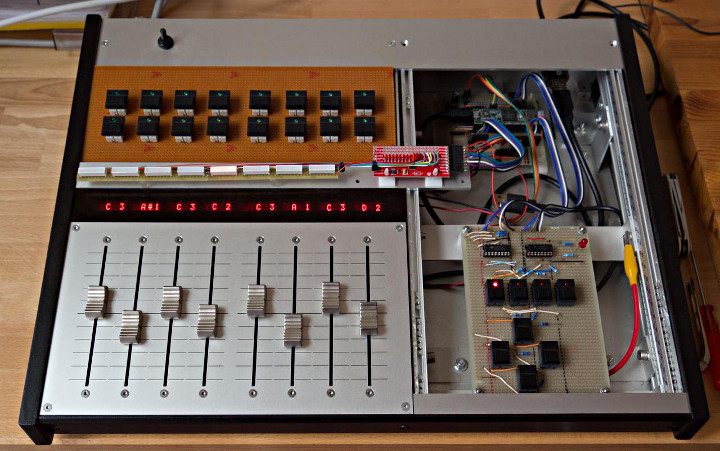The panel uses L293B H-bridges to drive the motors.  The fader wipers and touchtracks are connected directly to the Teensy. Each faders has it’s own PID control using 12 bit PWM at 14648.437 Hz. A second Teensy is reading the values from the faders via I2C and shows the raw values on the LED displays.

This project makes use of the ResponsiveAnalogRead.  Gerrit notes that he’s found the performance to be pretty good.  The faders can move fast and he’s been able to get very little overshoot, even at high speed.

## Teenstrument64-LC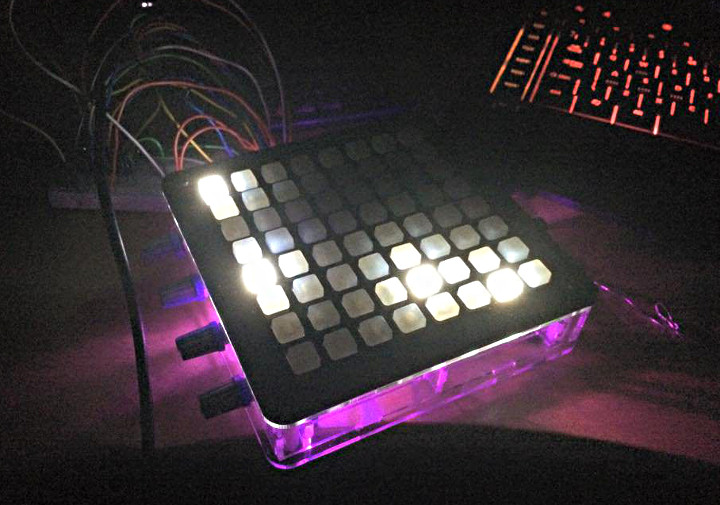This device features 3 instruments on 3 MIDI channels as well as a 32 step sequencer for each instrument.  It takes advantage of the native Teensy USB MIDI stack and also connects to an iPad using a USB camera connection kit.

## LED Wall Visualizer

James Best a very cool wall mounted LED music visualizer that lights up his room more than the over-head lights do.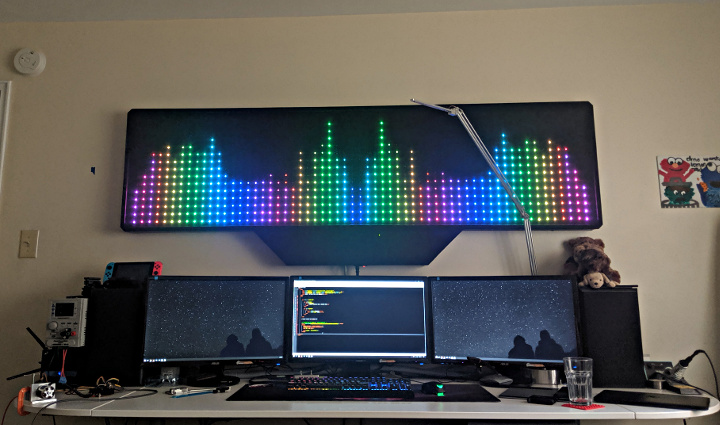Armed with blue tape and some hand tools, James got to work mounting 900 WS2812 LEDs.  After a miss-step with some Gaffers tape and some sanding, the adhesive on the LED strips adhered nicely to the wooden base.  The original Arduino didn’t have quite the processing power needed for the project, but the Teensy 3.2 with built in Direct Memory Access (DMA) was up to the task.  The final enhancement to the project was a diffuser to help hide the internal components.

## Dandelion Hunter

Arduino “having11” Guy created an autonomous robot to hunt down and destroy dandelions.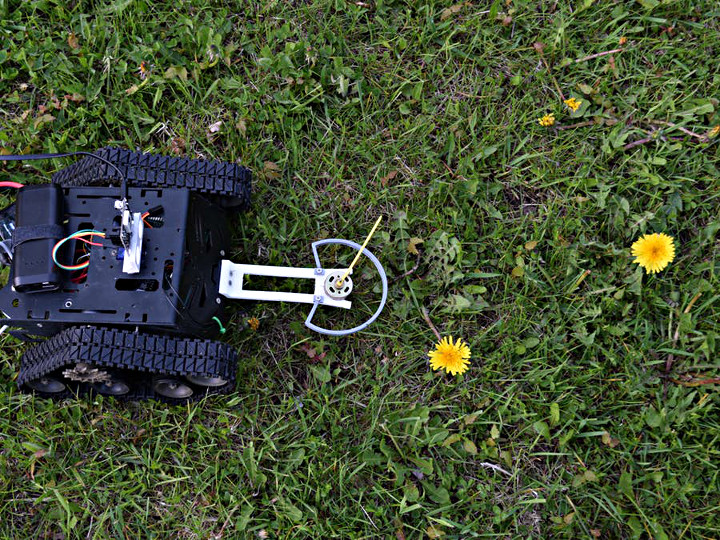After being frustrated a dandelions out pacing his grass between mows,  Arduino Guy (who also does project consulting for) built a robot using a Devastator Tank Cassis from DFRobots, a Pixy2 Camera, and a Teensy 3.5, to roam a yard to identify dandelions and chop them down.

Additional details as wells as the code is available on this Hackster.io project page.

## High Precision Sine Wave Synthesis Using Taylor Series

Normally sine waves are generated on microcontrollers using a table lookup.Lookup tables are perfect when wavelength happens to be an exact multiple of the sample rate, because you never actually need to know the values in between the table’s stored points.

But if you want to generate waveforms at any frequency without changing your sample rate, you end up needing points on the waveform that are between two entries in the table.  Four approaches are possible.

1. Use the prior point, even if the next point would have been better
2. Use the prior or next point, whichever is closer
3. Use both nearest points with linear interpolation
4. Use 3 or more nearest points, with spline or other non-linear interpolation

With any of these, larger lookup tables give better accuracy.  Since sine waves have symmetry, some programmers choose to store only 1/4 of the waveform and add slight overhead to map the other 3 quadrants onto the smaller table.

The Teensy Audio Library uses approach #3 for normal sine wave synthesis.  The vast majority of sine wave examples in the Arduino ecosystem use approach #1.

If you want a sine wave with extremely low distortion, where 16 or 20 or even 24 bits are within +/- 1 from an ideal sine wave, you would need an extremely large table!

Ideally, you’d want to be able to very rapidly compute an accurate sine value for any 32 bit resolution phase angle, so your samples always line up to an ideal sine wave.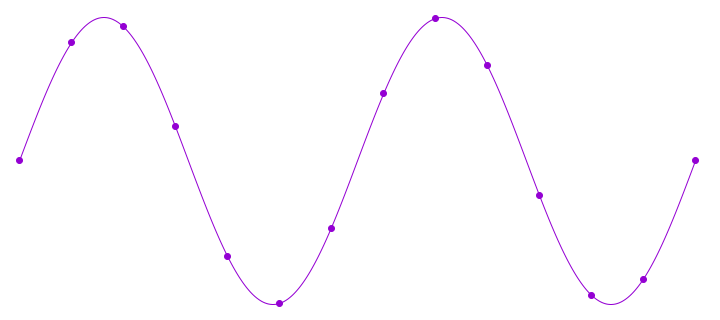Sine can be computed using Taylor series approximation.  The formula is: (where x is the angle, in radians)

sin(x) = x – (x^3)/3! + (x^5)/5! – (x^7)/7! + (x^9)/9! – (x^11)/11! + ….

This series goes on forever, but each extra terms makes the approximation rapidly converge to the true value.  In doing quite a lot of testing, I discovered the C library function on Linux for sin() uses this approximation, to only the (x^7)/7! term.  I also found a few sites talking about going to the (x^9)/9! for “professional quality” audio.

One nice advantage of cutting off the Taylor series with on of the subtracted powers (3, 7, 11, etc) is the tiny remaining error will always be slightly less than the true ideal sine value.  This means the final result does not need be checked for greater than 1.00000 and rounded down to fit into the maximum value of an integer.

If you’re still reading by this point, you’re probably shaking your head, thinking this couldn’t possibly be practical in a microcontroller.  That’s a complex equation with floating point numbers, and huge values in x^11 and 11!, since 11 factorial happens to be 39916800.

However, this Taylor series equation can be computed very efficiently, by exploiting the Cortex-M4 DSP extension instructions and bit shift operations, where the phase angle from 0 up to 2π is mapped from 0x00000000 to 0xFFFFFFFF.

The code I’m sharing here implements this equation to the (x^11)/11! term using 32 bit integers, using only 12 multiply instructions, which execute in a single cycle on Cortex-M4.  The add & subtract take zero CPU time, since those multiply instructions also come in flavors that do a multiply-and-accumulate, either positive or negative accumulate.

The Cortex-M4 multiplies perform a 32×32 to 64 bit multiply, and then discard the low 32 bits, with proper round off.  That turns out to be exactly the right thing for managing the huge values of x raised to an increasing power, and the huge numbers of the factorials.  Since those divisions are by constants, it’s possible to multiply by the reciprocal to get the same effect.

So, here’s is the optimized code:

https://github.com/PaulStoffregen/Audio/blob/master/synth_sine.cpp#L75

```// High accuracy 11th order Taylor Series Approximation
// input is 0 to 0xFFFFFFFF, representing 0 to 360 degree phase
// output is 32 bit signed integer, top 25 bits should be very good
static int32_t taylor(uint32_t ph)
{
int32_t angle, sum, p1, p2, p3, p5, p7, p9, p11;

if (ph >= 0xC0000000 || ph < 0x40000000) {
angle = (int32_t)ph; // valid from -90 to +90 degrees
} else {
angle = (int32_t)(0x80000000u - ph);
}
p1 =  multiply_32x32_rshift32_rounded(angle << 1, 1686629713);
p2 =  multiply_32x32_rshift32_rounded(p1, p1) << 3;
p3 =  multiply_32x32_rshift32_rounded(p2, p1) << 3;
sum = multiply_subtract_32x32_rshift32_rounded(p1 << 1, p3, 1431655765);
p5 =  multiply_32x32_rshift32_rounded(p3, p2) << 1;
sum = multiply_accumulate_32x32_rshift32_rounded(sum, p5, 286331153);
p7 =  multiply_32x32_rshift32_rounded(p5, p2);
sum = multiply_subtract_32x32_rshift32_rounded(sum, p7, 54539267);
p9 =  multiply_32x32_rshift32_rounded(p7, p2);
sum = multiply_accumulate_32x32_rshift32_rounded(sum, p9, 6059919);
p11 = multiply_32x32_rshift32_rounded(p9, p2);
sum = multiply_subtract_32x32_rshift32_rounded(sum, p11, 440721);
return sum <<= 1;
}
```

On top of the 12 cycles for multiplies, there’s a few bit shifts, and a quick conditional test which subtracts from a constant.  That’s necessary because the Taylor series approximation applies only if the angle is between -pi/2 to +pi/2.  For the other half of the sine wave, that subtract maps back into the valid range, because the sine wave has symmetry.

This function takes a 32 bit angle, where 0 represents 0 degrees, and 0xFFFFFFFF is just before 360 degrees.  So the input is perfect for a DDS phase accumulator.  The output is a 32 bit signed integer, where 0x7FFFFFFF represents an amplitude of +1.0, and 0x80000001 represents -1.0.

This code will never return 0x80000000, so you don’t need to worry about that case.

I did quite a lot of testing while working out these constants and the bit shifts for correct numerical ranges.  I believe the top 25 bits are “perfect”.  Six of the low 7 bits are very close, but the approximation does diverge slightly as the angle approaches pi/2 magnitude.  The LSB is always zero, since the computation needs to have extra overhead range to accommodate values representing up to ~1.57 (pi/2) before the latter terms converge to the final accurate value.

For 8 bit AVR, this approach probably isn’t practical.  It probably isn’t practical on Cortex-M0+ either, since there’s no 32×32 multiply with 64 bit result.  Cortex-M3 does have such a multiply, but not in the convenient version that rounds off and discards the low 32 bits.  On Cortex-M4, this code runs very fast.  In fact, when executing at 100 MHz or faster, it might even rival the table lookup, since non-sequential flash accesses (for the table) usually involve a few wait states for a cache miss.  Then again, this code does have 6 integer constants, for the conversion to radians and the factorial coefficients… and depending on compiler flags and flash caching behavior, loading those 6 constants might be the slowest part of this algorithm?

I’m sure most people will still use table lookups.  Linear interpolation between the nearest 2 table entries is fast and gives a result good enough for most applications.  Often a large table is also works well enough, without interpolation.  But I wanted to take a moment to share this anyway, even if it is massively overkill for most applications.  Hope you find it interesting.

UPDATE: Josy Boelen mentioned alternate forms for Taylor series approximation which require fewer multiplies.  Whether these could also be optimized with the M4 DSP extension instructions (not keeping full 64 bit resolution at every step) could be a really interesting future project…

This article was originally published in January 2016 (archive.org link) on the DorkbotPDX site.  Since then, the DorkbotPDX blog section has vanished.  I’m reposting it here with slight edits and a couple waveform plots, to preserve the info, and also because Michael Field recently asked for an article about these sorts of numerical approximations (which are rarely given as highly optimized fixed-point source code).

## Mega/Due Shield Breakout Board

Daniel Gilbert (Tall Dog on Tindie) has developed a breakout board that let’s you easily use Arduino shields  with the Teensy 3.5 or Teensy 3.6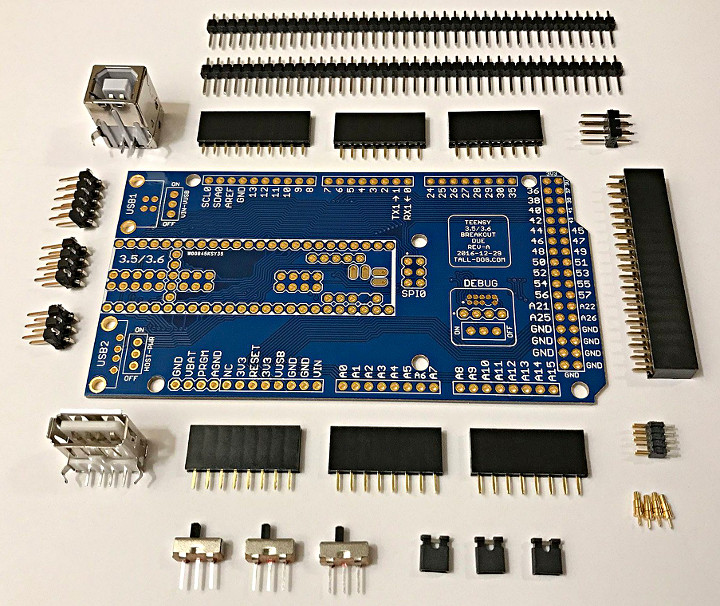This convenient board includes all parts needed to assemble a breakout board that allows you to connect a Teensy 3.5 or Teensy 3.6 to shields designed for the Arduino Due and Mega.  It features switches to select between USB or external power as one to set the US host port’s power mode (used for the Teensy 3.6).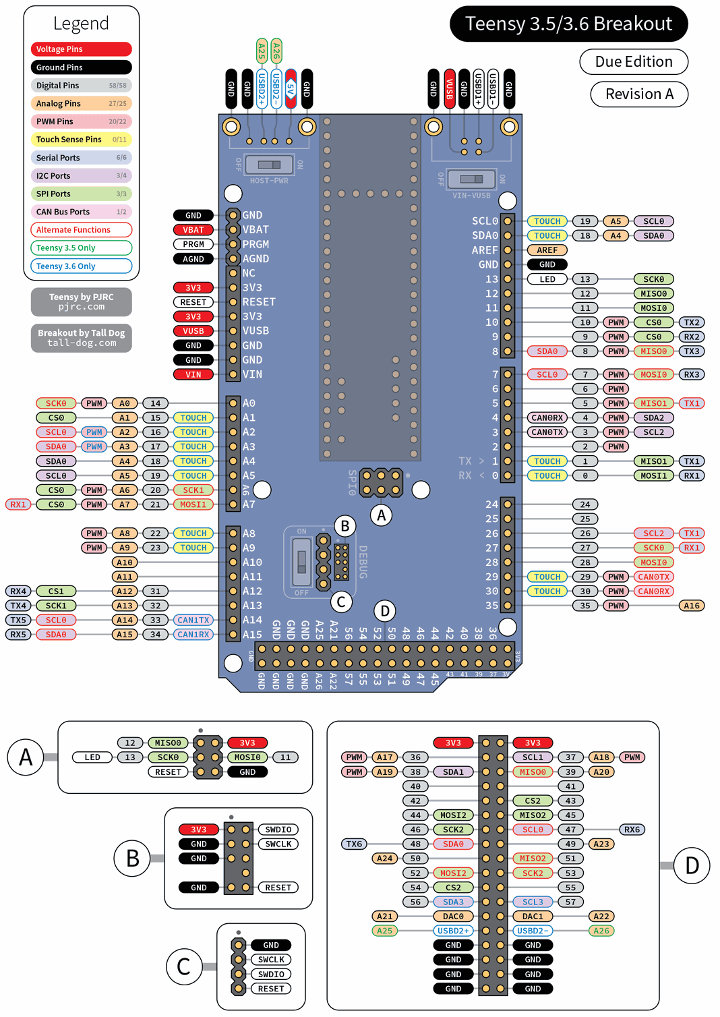## USB MIDI to 12 Gate and 16 Control Voltage Outputs

Sebastian Tomczak has improved his USB MIDI device from 8 gate and 16 CV outputs to 12 gate and 16 CV outputs.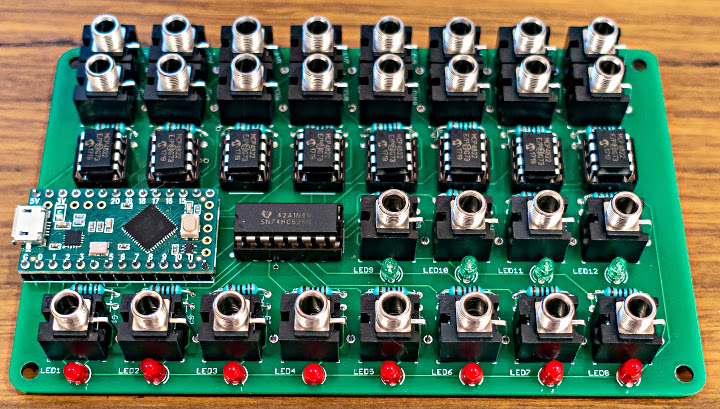This handy device has 16 control voltage (CV) outputs and 12 gate outputs.  USB MIDI channels 1 – 8 are mapped to CV outputs 1 – 16 for pitch and velocity, and gates 1 – 8 for note on and off events. Gates 9 – 12 are mapped to note on and off events only on channel 9, and also will send a sync and transport signal based on MIDI clock messages if received.

This is a new version of of a project we previously covered.

Schematics and a build guide are published on Sebastian’s blog.

Code for the project is available on GitHub.